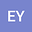•We introduce $$\oplus$$calculus and $$\otimes$$calculus for intuitionistic fuzzy values and prove some basic theorems by using multiplicative calculus which has useful tools to represent the concepts of introduced calculi. Besides, we construct some isomorphic mappings to interpret the relationships between $$\oplus$$calculus and $$\otimes$$calculus. This paper reveals also new calculi for fuzzy sets in particular.